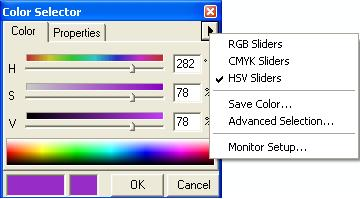English

# How To: Convert ArcView 3x HSV colors to ArcMap HSV equivalents

## Summary

Instructions provided describe how to convert ArcView 3x HSV colors to ArcMap HSV equivalents. The conversion of HSV (hue, saturation and value) in ArcView 3x to values in ArcMap can be calculated using the formulas below.

## Procedure

The ArcView HSV values are on a scale from 0 to 255. For example, a rich shade of purple in ArcView has HSV values of 200, 200, 200. These values, when entered in ArcMap custom colors, yield black. To convert them, perform the following:

1. The Hue value in ArcMap is scaled from 0 to 360, in degrees. Take the ArcView value, 200, divide by 255, and multiply by 360.

`Code:200/255 x 360 = 282 `

2. The Saturation and Value in ArcMap are percentages. Take the ArcView value, 200, divide by 255, and multiply by 100%.

`Code:200/255 x 100% = 78% `

In the example, both Saturation and Value are the same, so in ArcMap, both values are 78%.
3. In ArcMap, use the HSV style sliders to enter the HSV values.